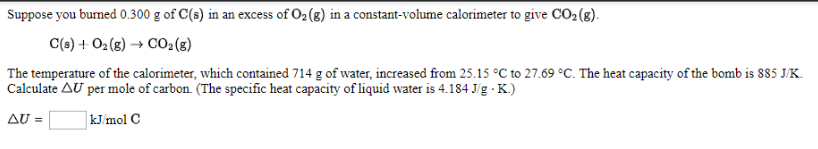# Suppose you burned 0.300 g of C(s) in an excess of O2(g) in a constant-volume calorimeter to give CO2(g). C(s) + O2(g) → CO2(g) The temperature of the calorimeter, which contained 714 g of water, increased from 25.15 degrees C to 27.69 degrees C. The heat capacity of the bomb is 885 J/K. calculate ∆U per mole of carbon. (The specific heat capacity of liquid water is 4.184 J/g . K.)